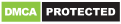All You Need To Know About Earning First \$100 from Google Adsense Visit Post

### The Future World : A train which runs inside Earth's core

A gravity train is a theoretical means of transportation intended to go between two points on the surface of a sphere, following a straight tunnel that goes directly from one point to the other through the interior of the sphere.

In a large body such as a planet, this train could be left to accelerate using just the force of gravity, since, during the first half of the trip (from the point of departure until the middle), the downwards pull towards the center of gravity would pull it towards the destination. During the second half of the trip, the acceleration would be in the opposite direction relative to the trajectory, but (ignoring the effects of friction) the speed acquired before would be enough to cancel this deceleration exactly (so that the train would reach its destination with speed equal to zero).

In reality, there are two reasons gravity trains do not exist. First, the transit shown in the illustration would pierce the Earth's mantle and traverse a region where rock is more fluid than solid. No materials are known that would withstand the tremendous heat and pressure in the inner core. Temperature is estimated as 5,700 K (5,430 °C; 9,800 °F), and pressure as high as about 330 to 360 gigapascals (3,300,000 to 3,600,000 atm). Secondly, frictional losses would be significant. Rolling friction losses could be reduced by using a magnetically levitated train. However, unless all air is evacuated from the tunnel, frictional losses due to air resistance would render the gravity train unusable. Evacuating the atmosphere to make it a vactrain would eliminate this drag but would require additional power. Such objections would not apply for solid planets and moons that do not have an atmosphere.

#### Where does this concept origin ?

In the 17th century, British scientist Robert Hooke presented the idea of an object accelerating inside a planet in a letter to Isaac Newton. A gravity train project was seriously presented to the Paris Academy of Sciences in the 19th century. The same idea was proposed, without calculation, by Lewis Carroll in 1893 in Sylvie and Bruno Concluded. The idea was rediscovered in the 1960s when physicist Paul Cooper published a paper in the American Journal of Physics suggesting that gravity trains be considered for a future transportation project.

#### What are the mathematical considerations behind it ?

The gravity train has several curious properties.All straight-line gravity trains on a given planet take exactly the same amount of time to complete a journey (that is, no matter where on the surface the two endpoints of its trajectory are located). For Earth, this time would equal 2530.30 seconds (nearly 42.2 minutes) if it were a perfect sphere and of uniform density.

The time of a trip depends only on the density of the planet and the gravitational constant (except when travelling at a significant proportion of the speed of light).

The maximum speed is reached at the middle point of the trajectory. For a train that goes directly through the center of the Earth, this maximum speed is about 7,900 metres per second (28440 km/h).The Time period of this simple harmonic motion is 84 minutes approximately.The derivation assumes that the mass is distributed homogeneously throughout the earth. The shortest time tunnel through a homogeneous earth is a Hypocycloid.